﻿ 基于混合元胞自动机法的结构拓扑优化软件平台开发 Software Platform Development for Structural Topology Optimization Based on Hybrid Cellular Automata

Mechanical Engineering and Technology
Vol. 08  No. 02 ( 2019 ), Article ID: 29809 , 7 pages
10.12677/MET.2019.82014

Software Platform Development for Structural Topology Optimization Based on Hybrid Cellular Automata

Kang Gui, Jianglin Zhang, Zhong He, Zefeng Sheng, Xiaolei Deng*

Key Laboratory of Air-Driven Equipment Technology of Zhejiang Province, Quzhou University, Quzhou Zhejiang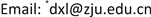Received: Mar. 28th, 2019; accepted: Apr. 15th, 2019; published: Apr. 22nd, 2019ABSTRACT

The theory and method of structural topology optimization of cellular automata are maturing and perfecting, and are gradually applied in practical engineering. This paper expounds the principle of hybrid cellular automata method, analyses the technical framework and development environment of software development, and develops a software platform for structural topology optimization design of hybrid cellular automata method based on MATLAB and ANSYS, so as to reduce the difficulty of structural topology optimization by designers using hybrid cellular automata method. Finally, the validity of the software is verified by a classical two-dimensional numerical example.

Keywords:Hybrid Cellular Automata, Topology Optimization, Software Platform Development1. 引言

2. 混合元胞自动机法基本原理简介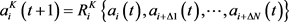(1)

${a}_{i}\left(t\right)=\left[\begin{array}{l}{x}_{i}\left(t\right)\\ {S}_{i}\left(t\right)\end{array}\right]$ (2)

$U=\underset{i=1}{\overset{N}{\sum }}{U}_{i}{v}_{i}$ (3)

${U}_{i}=\frac{1}{2}{\sigma }^{\text{T}}\epsilon$ (4)

xy，yz，xz三个面上的剪应力。

$\left\{\begin{array}{l}\mathrm{min}|{e}_{i}|=|{\stackrel{¯}{U}}_{i}-{U}^{*}|\\ \text{s}\text{.t}\text{.}\text{\hspace{0.17em}}\text{\hspace{0.17em}}0<{x}_{\mathrm{min}}\le {x}_{i}\le 1,\text{\hspace{0.17em}}\text{\hspace{0.17em}}\text{\hspace{0.17em}}\text{\hspace{0.17em}}i=1,2,\cdots ,N\end{array}$ (5)

3. 混合元胞自动机法结构拓扑优化设计软件开发

3.1. 软件分析流程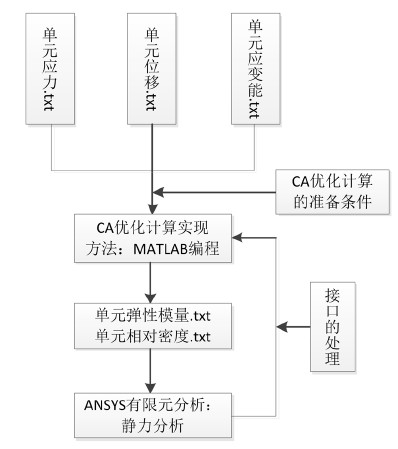Figure 1. Platform flowchart

3.2. 技术构架

3.3. 开发环境

3.4. 软件主界面

HCAM结构拓扑优化设计软件界面主要包括参数子界面选择栏、结果显示选择栏、结果显示区和工作区等，结果显示区显示用户选择查看的结果，平台主界面、材料参数和边界条件设置界面如图2所示。

3.5. 软件功能实现

HCAM结构拓扑优化设计软件平台的模块可划分为四个部分：一是参数输入模块，包括样机结构参数输入、环境参数输入、边界条件输入、MATLAB文件调用等；二是分析模块，相应的设置完成后调用后台ANSYS程序进行优化分析计算；三是结果查询模块，进行结果的查询分析；四是数据管理模块，进行所有分析数据包括结果等在内的管理和更新。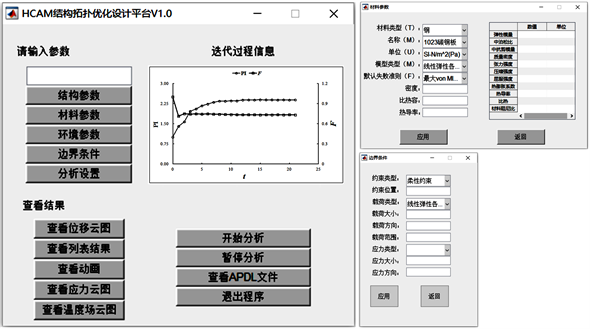Figure 2. Platform interface

4. 案例分析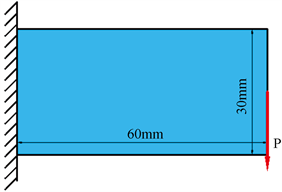Figure 3. TOCS standard test component base structure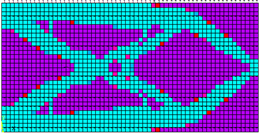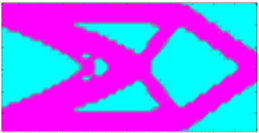(a) HCAM的拓扑优化构形 (b) 文献  的拓扑优化构形

Figure 4. Results comparison of different optimization algorithms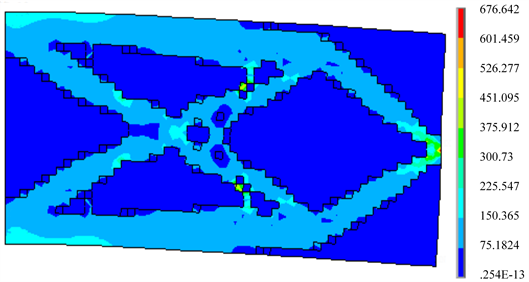Figure 5. Stress nephogram of final topological optimization configuration

5. 结论

Software Platform Development for Structural Topology Optimization Based on Hybrid Cellular Automata[J]. 机械工程与技术, 2019, 08(02): 111-117. https://doi.org/10.12677/MET.2019.82014

1. 1. Andrés, T., Patel, N.M., Kaushik, A.K., et al. (2004) Hybrid Cellular Automata: A Biologically-Inspired Structural Optimization Technique. 10th AIAA/ISSMO Multidisciplinary Analysis and Optimization Conference, New York, Virginia, 30 August-1 September 2004, 2844-2858.

2. 2. Andrés, T., Wilson, I.Q., Neal, M.P., et al. (2005) Hybrid Cellular Automata with Local Control Rules: A New Approach to Topology Optimization Inspired by Bone Functional Adaptation. 6th World Congresses of Structural and Multi-disciplinary Optimization Rio de Janeiro, 30 May-3 June 2005, Rio de Janeiro, 1-11.

3. 3. Andrés, T., Patel, N.M., Niebur, G.L., et al. (2006) Topology Optimization Using a Hybrid Cellular Automaton Method with Local Control Rules. Journal of Mechanical Design, 128, 1205-1216. https://doi.org/10.1115/1.2336251

4. 4. Zakhama, R., Abdalla, M.M., Smaoui, H., et al. (2009) Multigrid Implementation of Cellular Automata for Topology Optimization of Continuum Structures. Computer Modeling in Engineering and Sciences (CMES), 51, 1-24.

5. 5. 郭连水, Punit, B., John, E.R. 一种大变形多空间域连续体结构拓扑优化方法[J]. 北京航空航天大学学报, 2009, 35(2): 227-230. https://doi.org/10.1590/S1517-97022009000200001

6. 6. Guo, L.S., Andrés, T., Penninger, C.L., et al. (2011) Strain-Based Topology Optimisation for Crashworthiness Using Hybrid Cellular Automata. International Journal of Crashworthiness, 13, 239-252. https://doi.org/10.1080/13588265.2010.544892

7. 7. Du, Y., Chen, D., Xiang, X., et al. (2013) Topological Design of Structures Using a Cellular Automata Method. CMES: Computer Modeling in Engineering and Sciences, 94, 53-75.

8. 8. 张一雨, 郑百林, 吴一帆, 等. 鸟撞工况下HCA动态拓扑优化整级叶片的数值模拟[J]. 推进技术, 2019, 40(2): 431-440.

9. 9. 周珍珍. 基于混合元胞自动机方法的结构拓扑优化研究[D]: [硕士学位论文]. 武汉: 华中科技大学, 2009.

10. 10. 高扬. 基于.NET平台的三层架构软件框架的设计与实现[J]. 计算机技术与发展, 2011, 21(2): 77-80, 85.

NOTES

*通讯作者。